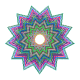# The Coaster Maker by Shiny

The word you invented is well formed and could be used in the Italian language (The Accademia della Crusca regarding to the word “Petaloso”, recently invented by an eight-year-old boy)

Are you tired of your old coasters? Do you like to make things by your own? Do you have a PC and a printer at home? If you answered yes to all these questions, just follow these simple instructions:

• Install R and RStudio in your PC
• Open RStudio and create a new Shiny Web App multiple file (ui.R/server.R)
• Substitute sample code of each file by the code below
• Press Run App
• Press buttom Get your coaster! until you obtain a image you like
• Print the image
• Cut out the image
• Place on the coaster your favorite drinking

These are some examples:

This is the code of ui.R

```#
# This is the user-interface definition of a Shiny web application. You can
# run the application by clicking 'Run App' above.
#
# Find out more about building applications with Shiny here:
#
#    http://shiny.rstudio.com/
#
library(shiny)
shinyUI(fluidPage(
titlePanel("The coaster maker"),
sidebarLayout(
sidebarPanel(
#helpText(),

# adding the new div tag to the sidebar
tags\$p("This coasters are generated by hypocycloid curves.The curve is formed by the locus of a point,
attached to a circle, that rolls on the inside of another circle.
In the curve's equation the first part denotes the relative position between the two circles,
the second part denotes the rotation of the rolling circle.")),
HTML(
),
mainPanel(
plotOutput("HarmPlot")
)
)
))
```

This is the code of server.R

```# This is the server logic of a Shiny web application. You can run the
# application by clicking 'Run App' above.
#
# Find out more about building applications with Shiny here:
#
#    http://shiny.rstudio.com/
#
library(shiny)
library(ggplot2)
CreateDS = function ()
{
t=seq(-31*pi, 31*pi, 0.002)
a=sample(seq(from=1/31, to=29/31, by=2/31), 1)
b=runif(1, min = 1, max = 3)
data.frame(x=(1-a)*cos(a*t)+a*b*cos((1-a)*t), y=(1-a)*sin(a*t)-a*b*sin((1-a)*t))
}
shinyServer(function(input, output) {
dat<-reactive({if (input\$rerun) dat=CreateDS() else dat=CreateDS()})
output\$HarmPlot<-renderPlot({
ggplot(dat())+
geom_point(data=data.frame(x=0,y=0), aes(x,y), color=rgb(rbeta(1, .5, .5), rbeta(1, .5, .5), rbeta(1, .5, .5)) , shape=19, fill="yellow", size=220)+
geom_polygon(aes(x, y), fill=rgb(rbeta(1, 2, 2), rbeta(1, 2, 2), rbeta(1, 2, 2))) +
theme(legend.position="none",
panel.background = element_rect(fill="white"),
panel.grid=element_blank(),
axis.ticks=element_blank(),
axis.title=element_blank(),
axis.text=element_blank())
}, height = 500, width = 500)
})
```

## 1 thought on “The Coaster Maker by Shiny”

1.Yuriy Chistyakov says:

this lines:
HTML(”